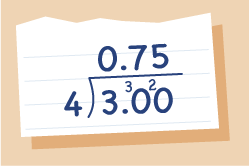Everyday maths 2 (Wales)

Start this free course now. Just create an account and sign in. Enrol and complete the course for a free statement of participation or digital badge if available.

# 9.2 Changing a fraction to a percentage

There are two ways you can do this.

## Method 1

To change a fraction into a percentage, multiply it by (essentially, you are just multiplying the top number by 100 and the bottom number will stay the same).

### Example: Change three divided by four into a percentage

×  =

This cancels to = 75%

Note: Remember anything over 1 is a whole number. If you do not end up with a 1 on the bottom, you will have to divide the top number by the bottom one to get your final answer.

## Method 2

Divide the top of the fraction by the bottom (to express the fraction as a decimal) and then multiply the answer by 100.

### Example: three divided by four = 3 ÷ 4 = 0.75Figure 12 Expressed as a decimal: 3 ÷ 4
• 0.75 × 100 = 75%

## Activity 22: Changing a fraction to a percentage

1. Express these fractions as percentages:

• a.

• b.

• c.

1.

• a.37.5%

• b.90%

• c.80%

Now you’ll look at changing a fraction to a decimal.#### Find out more

*Eligibility rules apply for financial support.